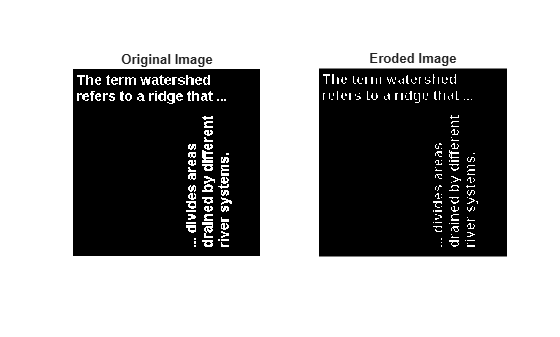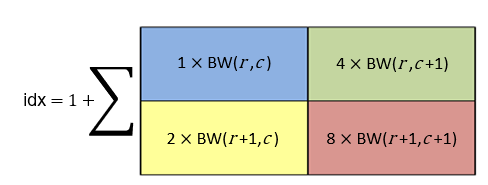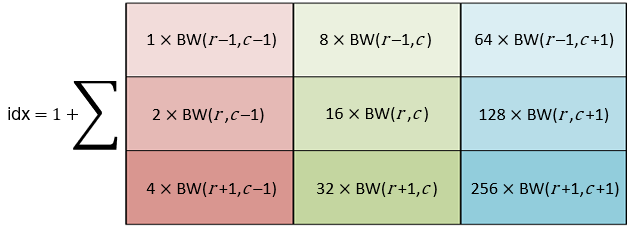# bwlookup

Nonlinear filtering using lookup tables

## Syntax

``J = bwlookup(BW,lut)``

## Description

example

````J = bwlookup(BW,lut)` performs a 2-by-2 or 3-by-3 nonlinear neighborhood filtering operation on binary image `BW`. The neighborhood processing determines an integer index value used to access values in lookup table `lut`. The fetched `lut` value becomes the pixel value in output image `J` at the targeted position.```

## Examples

collapse all

Construct the vector `lut` such that the filtering operation places a `1` at the targeted pixel location in the input image only when all four pixels in the 2-by-2 neighborhood of BW are set to `1`.

```lutfun = @(x)(sum(x(:))==4); lut = makelut(lutfun,2)```
```lut = 16×1 0 0 0 0 0 0 0 0 0 0 ⋮ ```

`BW1 = imread('text.png');`

Perform 2-by-2 neighborhood processing with 16-element vector `lut` .

`BW2 = bwlookup(BW1,lut);`

Show zoomed before and after images.

```figure; h1 = subplot(1,2,1); imshow(BW1), axis off; title('Original Image') h2 = subplot(1,2,2); imshow(BW2); axis off; title('Eroded Image') % 16X zoom to see effects of erosion on text set(h1,'Ylim',[1 64],'Xlim',[1 64]); set(h2,'Ylim',[1 64],'Xlim',[1 64]);```## Input Arguments

collapse all

Binary image to be transformed by the nonlinear neighborhood filtering operation, specified as a 2-D logical matrix or 2-D numeric matrix. For numeric input, any nonzero pixels are considered to be `1` (`true`).

Data Types: `single` | `double` | `int8` | `int16` | `int32` | `int64` | `uint8` | `uint16` | `uint32` | `uint64` | `logical`

Lookup table of output pixel values, specified as a 16- or 512-element vector. The size of `lut` determines which of the two neighborhood operations is performed. You can use the `makelut` function to create a lookup table.

• If `lut` contains 16 data elements, then the neighborhood matrix is 2-by-2.

• If `lut` contains 512 data elements, then the neighborhood matrix is 3-by-3.

Data Types: `single` | `double` | `int8` | `int16` | `int32` | `int64` | `uint8` | `uint16` | `uint32` | `uint64` | `logical`

## Output Arguments

collapse all

Output image, returned as a grayscale or binary image whose distribution of pixel values are determined by the content of the lookup table, `lut`. The output image `J` is the same size as the input image `BW` and the same data type as `lut`.

## Algorithms

collapse all

The first step in each iteration of the filtering operation performed by `bwlookup` entails computing the `index` into vector `lut` based on the binary pixel pattern of the neighborhood matrix on image `BW`. The value in `lut` accessed at `index`, `lut(index)`, is inserted into output image `J` at the targeted pixel location. This results in image `J` being the same data type as vector `lut`.

Since there is a 1-to-1 correspondence in targeted pixel locations, image `J` is the same size as image `BW`. If the targeted pixel location is on an edge of image `BW` and if any part of the 2-by-2 or 3-by-3 neighborhood matrix extends beyond the image edge, then these non-image locations are padded with 0 in order to perform the filtering operation.

The following figures show the mapping from binary 0 and 1 patterns in the neighborhood matrices to its binary representation. Adding 1 to the binary representation yields `index` which is used to access `lut`.

### 2-by-2 Neighborhood Lookup

For 2-by-2 neighborhoods, `length(lut)` is 16. There are four pixels in each neighborhood, and two possible states for each pixel, so the total number of permutations is 24 = 16.To illustrate, this example shows how the pixel pattern in a 2-by-2 matrix determines which entry in `lut` is placed in the targeted pixel location.

1. Create random 16-element `lut` vector containing `uint8` data.

```scurr = rng; % save current random number generator seed state rng('default') % always generate same set of random numbers lut = uint8( round( 255*rand(16,1) ) ) % generate lut rng(scurr); % restore```
```lut = 208 231 32 233 161 25 71 139 244 246 40 248 244 124 204 36```
2. Create a 2-by-2 image and assume for this example that the targeted pixel location is location `BW(1,1)`.

`BW = [1 0; 0 1]`
```BW = 1 0 0 1```
3. By referring to the color coded mapping figure above, the binary representation for this 2-by-2 neighborhood can be computed as shown in the code snippet below. The logical 1 at `BW(1,1)` corresponds to blue in the figure which maps to the Least Significant Bit (LSB) at position 0 in the 4-bit binary representation (,20= 1). The logical 1 at `BW(2,2)` is red which maps to the Most Significant Bit (MSB) at position 3 in the 4-bit binary representation (23= 8) .

```% BW(1,1): blue square; sets bit position 0 on right % BW(2,2): red square; sets bit position 3 on left binNot = '1 0 0 1'; % binary representation of 2x2 neighborhood matrix X = bin2dec( binNot ); % convert from binary to decimal index = X + 1 % add 1 to compute index value for uint8 vector lut A11 = lut(index) % value at A(1,1)```
```index = 10 A11 = 246```
4. The above calculation predicts that output image A should contain the value 246 at targeted position `A(1,1)`.

`A = bwlookup(BW,lut) % perform filtering`
```A = 246 32 161 231```

`A(1,1)` does in fact equal 246.

### 3-by-3 Neighborhood Lookup

For 3-by-3 neighborhoods, `length(lut)` is 512. There are nine pixels in each neighborhood, and two possible states for each pixel, so the total number of permutations is 29 = 512.

The process for computing the binary representation of 3-by-3 neighborhood processing is the same as for 2-by-2 neighborhoods.